# 什么是注意力机制？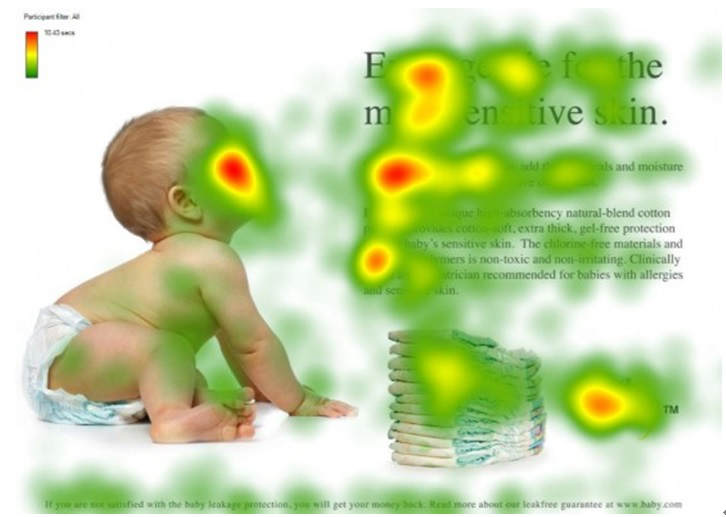# Encoder-Decoder 框架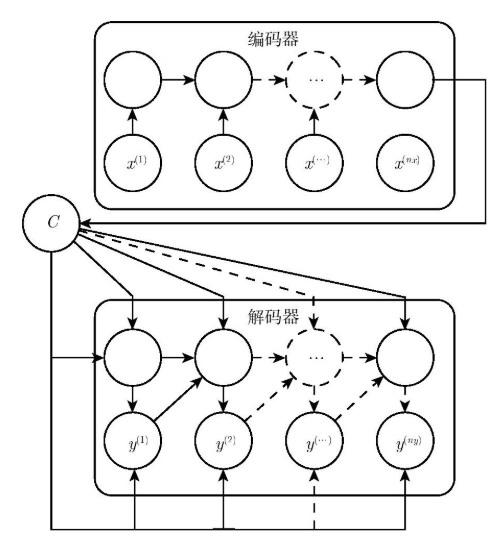$h_t = f(h_{t-1},x_t)\tag{1}$

$C=q(h_1,h_2,\cdots,h_{T_x})\tag{2}$

$C=q(h_1,h_2,\cdots,h_{T_x})=h_{T_x}\tag{3}$

$y_t=\arg \max P(y_t)=\prod_{t=1}^{T}p(y_t|y_1,y_2,\cdots,y_{t-1},C)\tag{4}$

$y_t=g(y_{t-1},s_{t-1},C)\tag{5}$

## 局限性

Encoder-Decoder 框架虽然应用广泛，但是其存在的局限性也比较大。其最大的局限性就是 Encoder 和 Decoder 之间只通过一个固定长度的语义向量 $C$ 来唯一联系。也就是说，Encoder 必须要将输入的整个序列的信息都压缩进一个固定长度的向量中，存在两个弊端：一是语义向量 C 可能无法完全表示整个序列的信息；二是先输入到网络的内容携带的信息会被后输入的信息覆盖掉，输入的序列越长，该现象就越严重。这两个弊端使得 Decoder 在解码时一开始就无法获得输入序列最够多的信息，因此导致解码的精确度不够准确。

# Attention 机制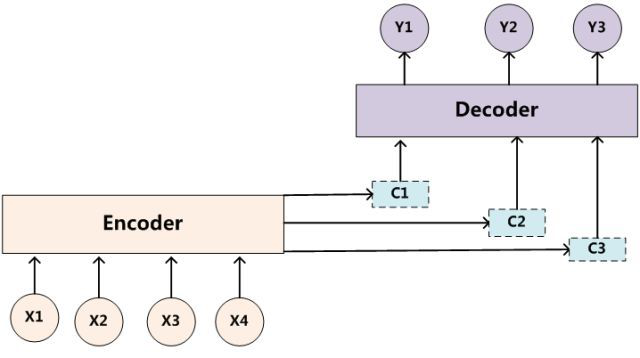$C_i=\sum_{j=0}^{T_x}{a_{ij}f(x_j)}\tag{6}$

$a_{ij}=\frac{exp(e_{ij})}{\sum_{k=1}^{T_x}exp(e_{ik})}\tag{7}$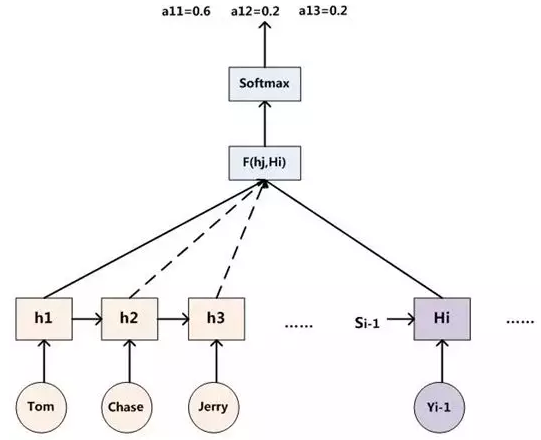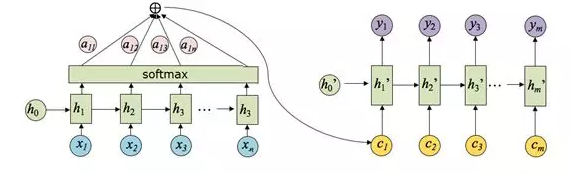# Attention 原理

Attention 机制的一个重点就是获得 attention value，即机器翻译中的语义编码 $C_i$。在上一节中我们知道该值是通过输入元素按照不同的权重参数组合而成的，所以我们可以将其定义为一个 attention 函数，比较主流的 attention 函数的机制是采用键值对查询的方式，其工作实质如下图所示：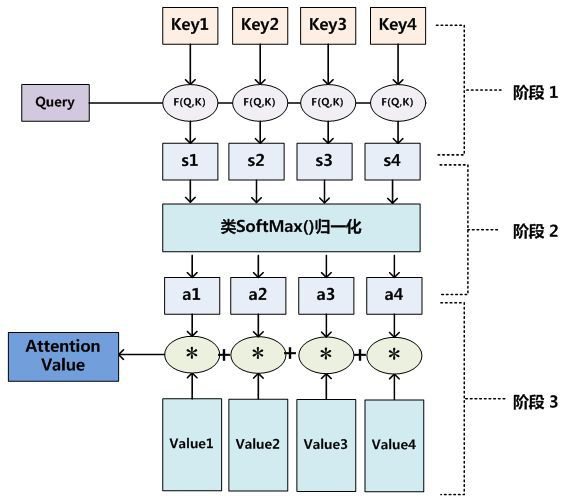Step 0：准备隐藏状态Step 1：得到每一个 Encoder 隐藏状态的得分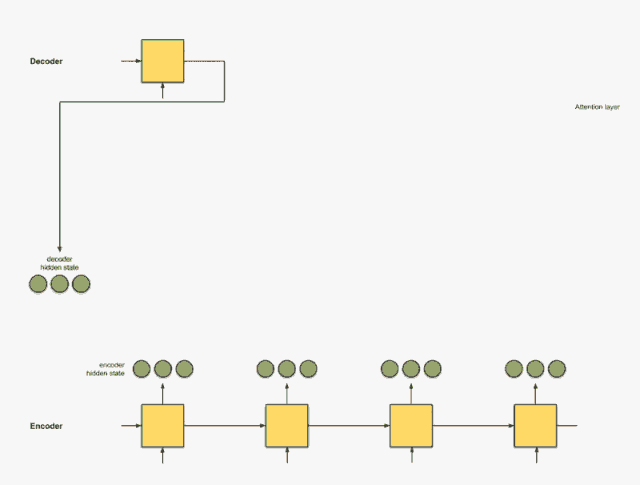decoder_hidden = [10, 5, 10]
encoder_hidden score
---------------------
[0, 1, 1]     15 (= 10×0 + 5×1 + 10×1, the dot product)
[5, 0, 1]     60
[1, 1, 0]     15
[0, 5, 1]     35

Step 2：将所有得分送入 softmax 层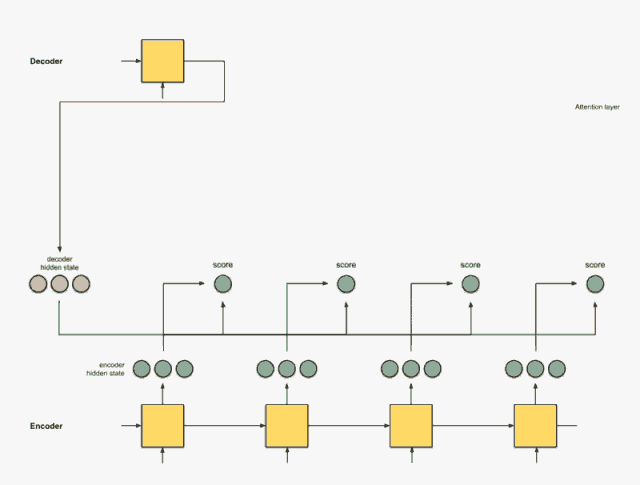encoder_hidden score score^
-----------------------------
[0, 1, 1]     15       0
[5, 0, 1]     60       1
[1, 1, 0]     15       0
[0, 5, 1]     35       0

Step 3：用每个 Encoder 的隐藏状态乘以 softmax 之后的得分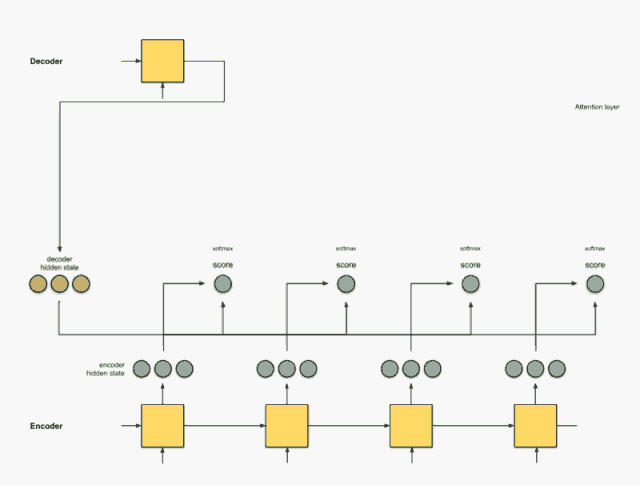encoder score score^ alignment
---------------------------------
[0, 1, 1]   15     0   [0, 0, 0]
[5, 0, 1]   60     1   [5, 0, 1]
[1, 1, 0]   15     0   [0, 0, 0]
[0, 5, 1]   35     0   [0, 0, 0]

Step 4：将所有对齐的向量进行累加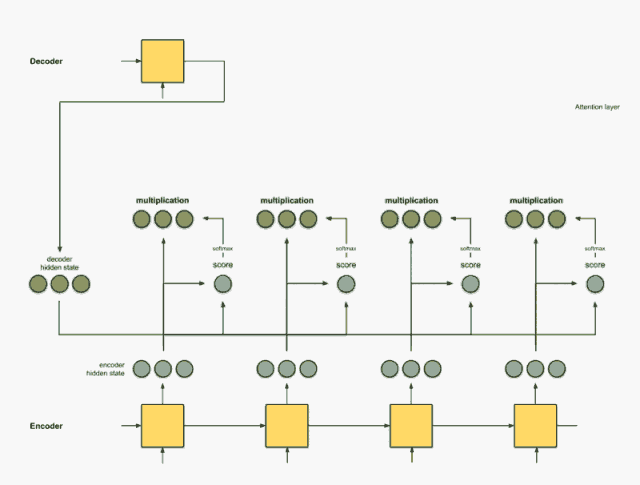Step 5：把上下文向量送到 Decoder 中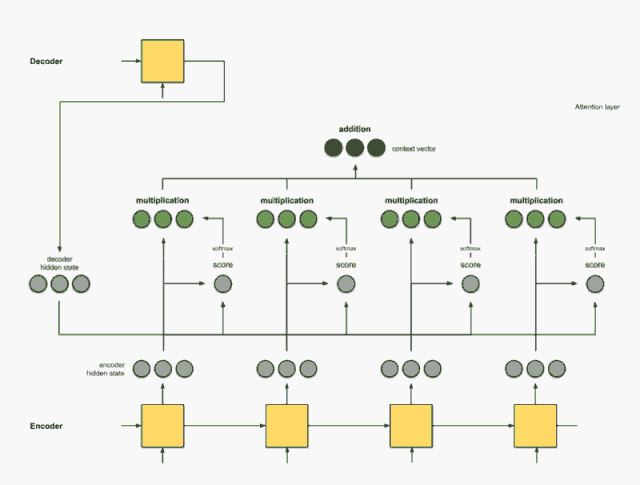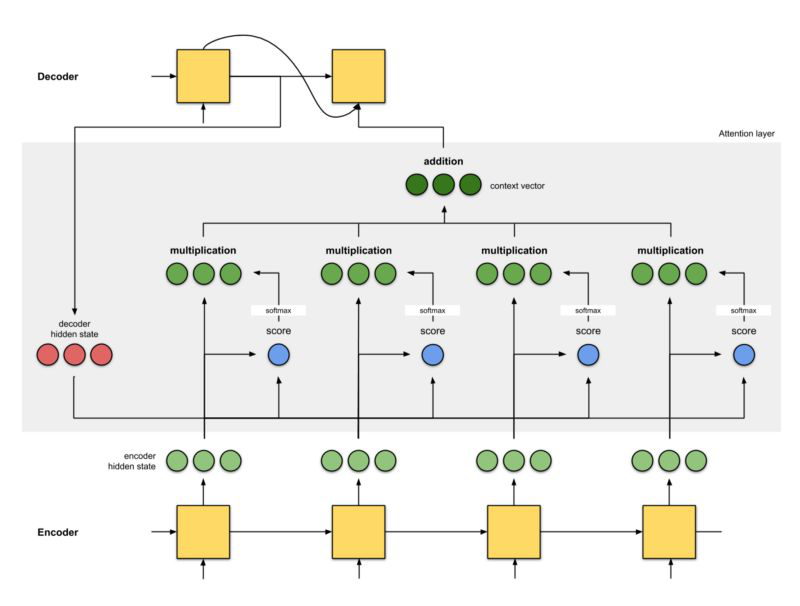# Attention 机制的优劣

• 一步到位的全局联系捕捉，且关注了元素的局部联系；attention 函数在计算 attention value 时，是进行序列的每一个元素和其它元素的对比，在这个过程中每一个元素间的距离都是一；而在时间序列 RNNs 中，元素的值是通过一步步递推得到的长期依赖关系获取的，而越长的序列捕捉长期依赖关系的能力就会越弱。

• 并行计算减少模型训练时间；Attention 机制每一步的计算都不依赖于上一步的计算结果，因此可以并行处理。

• 模型复杂度小，参数少

# 参考资料

posted @ 2019-06-17 09:50  Maple17  阅读(...)  评论(...编辑  收藏